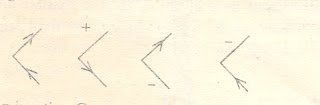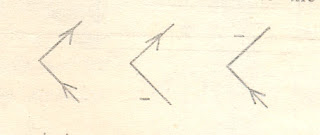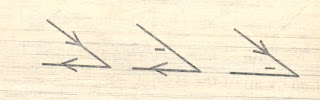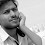## Friday, February 18, 2011

### Waltz Algorithm.

CONSTRAINT SATISFACTION – WALTZ ALGORIHTM

Many perceptual tasks appear to be highly complex . this is because the number of interpretations that can be assigned to individual components of an input is large and the number of combinations of those components also appears to be enormous. But a clear analysis well reveal that many do the combinations can not actually occur. These natural constraints can be exploited in the understanding process to reduce the complexity from unmanageable to manageable.

There are two important steps in the use of constraints in problem solving:

1. Analyze the problem domain to determine the actual constraints.

2. Solve the problem by applying a constraint satisfaction algorithm.

Consider for example a three dimensional line drawing . The analysis process is to determine the object described by the lines. The geometric relationships between different types of line junctions helped to determine the object typ

Three Dimentioal Polyhedral junction types.

In waltz’s algorithm, labels are assigned to lines of various types-say concave edges are produced by two adjacent toching surfaces which duce a concave (less than 180 Degrees) depth change .

Conversely, convex edges produce a convexly viewed depth (greater than 180 degrees), and a boundary edge outlines a surface that obstracts other objects.

To label a concave edge, a mints sign is used. Convex edges one labeled with a plus sign, and a right or left arrow is used to label the boundary edges. By restricting vertices to be the intersection of three object faces, it is possible to reduce the number of basic vertex types to only four : the L, the T , the Fork and the Arrow.

The L types Fork Types T types

Valid junction labels for three-dimensional shapes.

When a three-dimensional object is viewed from all possible positions, the four junction types, together with the valid edge labels, give rise to eighteen different permissible junction configurations as shown in figurre

Geometric constraints, together with a consistent labeling scheme, can simplify the object identification process. A set of labeling rules which facilitates this process can be developed for different classes of objects. The following rules will apply for many polyhedral objects.

1. The arrow should be directed to mark boundaries by traversing the object in a clockwise direction.

2. Unbroken lines should have the same lable assigned at both ends.

3. When a fork is labeled with a+ edge, it must have all three edges label as + , and

4. Arrow junctions which have aà label on both bard edges must also have a + label on the shaft.

These rules can be applied to a polygonal object as given in figureexample of object labeling.

Starting with any edge having an object face on its right, the external boundary is labeled with the à in a clockwise direction. Interior lines are then labeled with + or _ consistent with the ot

her labeling rules.

To see how waltz constraint satisfaction algorithm works, consider the image dr

awing of a pyramid as given in figure3 At the right side of the pyramid are all po

ssible labelings for the four u\junctions A, b, C and D.Using these labels as mutual constraints on connected junctions, permissible labels for the whole pyramid can be determined. The constraint satisfaction procedure works as follows.

Starting at an arbitrary junction, say A, a record of all permissible labels is made

for that junction. An adjacent junction is then chosen, say , B and labels which are

inconsistent with the line AB are then eliminated from the p

ermissible A and B lists. In this case, the line joining B can only be a +, - or an up

arrowà consequently, two of the possible

A labelings can be eliminated and the remainin

g areChoosing junction c next, we find that t

he BC constraints are

satisfied by all of the B and C lableings, so on reduction

is possible with this step. On the otherhand, the line AC must be labled as – or as an up-

left-arrow ß to be consistent. Therefore, an additional label for A can be eliminated to reduce the remainder to the following.This new restriction on a now permit the elimination of one B leabeling to maintain consistency. Thus, the permissible B leabelings remaining are nowThis reduction in turn, places a new restriction on BC, permitting the elimination of one C label, since BC must now be labeled as a + only. This leaves the remaining C labels as show in side diagram.

4. Moving now to junction d, we see that of the six possible D leadings, only three satisfy the BD constraint of a up or a down arrow. Therefore, the remaining permissible leabelings for d are nowContinuing with the above procedure, we see that further label eliminations are not possible since all constraints have been satisfied. This process is completed by finding the different combinations of unique lableings that can be assigned to the figure. An enumerations of the remaining label shows that its is possible to find only three different lableings.

1.2.